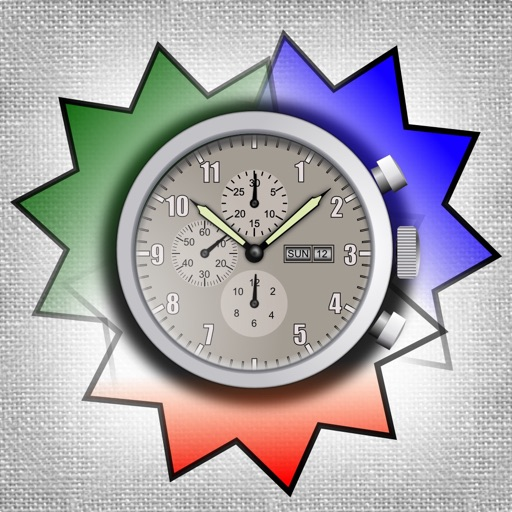## The Time Calculator+ is the best time calculator app in the store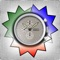# Time Calculator+

by Verosocial Studio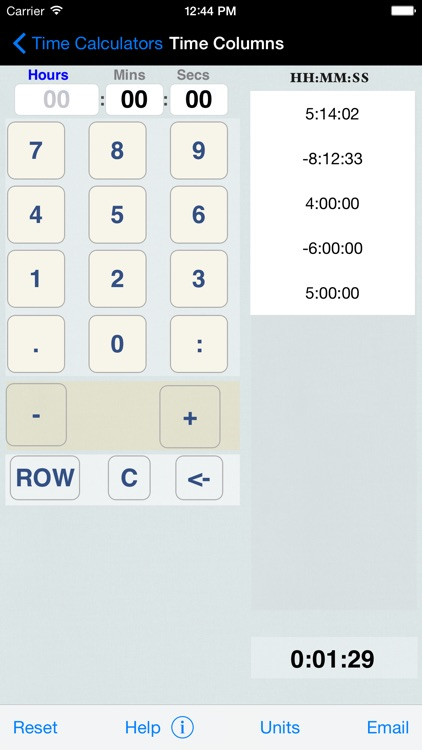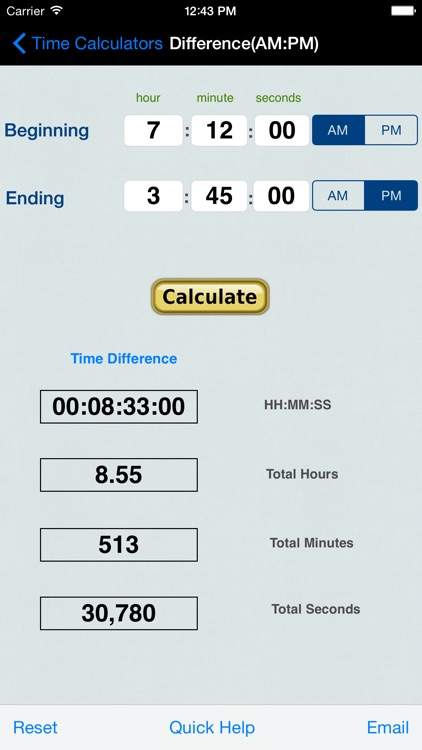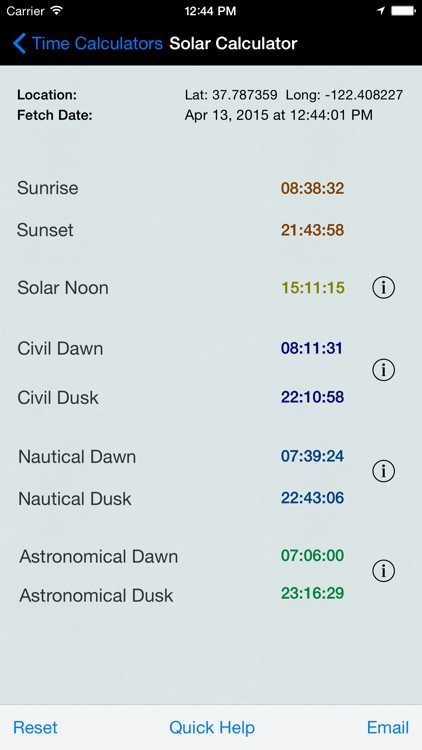The Time Calculator+ is the best time calculator app in the store. The app consists of a dozen date and time calculators in a single app, and is designed for both right-handed and left-handed people.Version
15.6
Rating
(7)
Size
19Mb
Genre
Last updated
June 6, 2019
Release date
July 25, 2011

### App Screenshots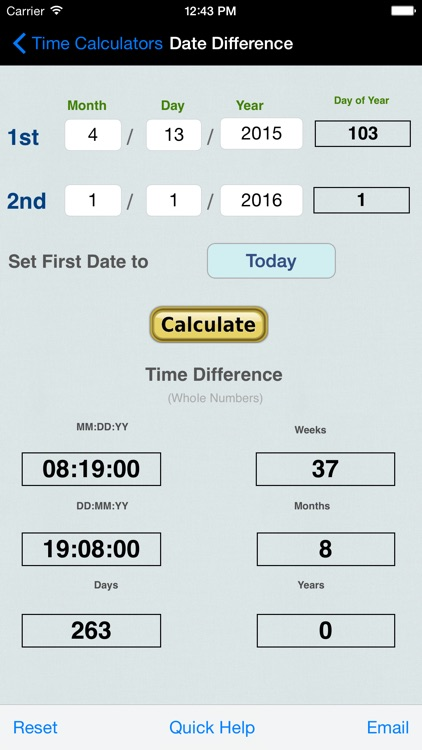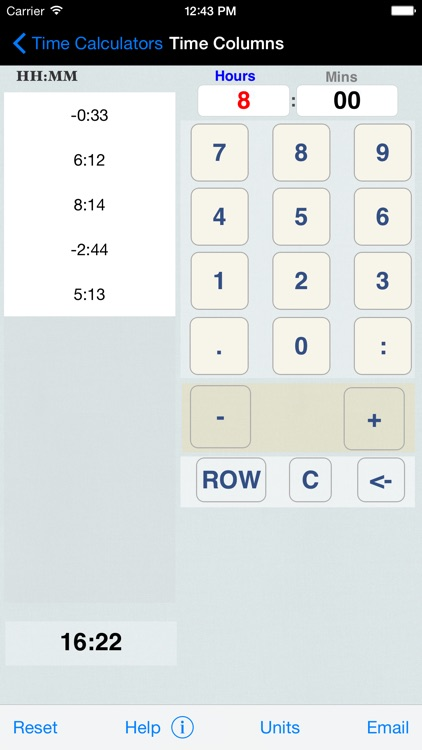### App Store Description

The Time Calculator+ is the best time calculator app in the store. The app consists of a dozen date and time calculators in a single app, and is designed for both right-handed and left-handed people.

Time calculator functions list

1) Time Columns (Days:Hours:Minutes:Seconds)
2) Time Columns (Hours:Minutes:Seconds)
3) Time Columns (Hours:Minutes)
3) Time Columns (Minutes:Seconds)
4) Multiply Time
5) Divide Time
6) Time Difference (24 Hr Clock)
7) Time Difference (AM:PM Clock)
8) Date Difference Calculator & Day of Year Converter (Julian Day)
9) Units of Time Conversion
10) Date Add and Subtract Calculator
11) Day of Year to Date Converter
12) Date to Day of Year Converter

EXTRA: Solar Day events for Sunrise/Sunset, Dawn, Dusk, and Noon.

The Time Columns Calculators use time expressions in a vertical list and applies addition and subtraction to produce the total as accumulated time. This can be sent as email and/or saved.

Example - using the Days:Hours:Minutes:Seconds - format

Accumulated Times:
1:08:03:00
-0:05:06:00
-0:09:00:00

Calculated Time:
0:17:57:00

Example - using the Hours:Minutes - format

Accumulated Times:
18:03
-05:06
-09:00

Calculated Time:
3:57

The Multiply Time Calculator multiplies an amount of time against a decimal number and converts the result to a time expression and the total in four different time units: Days, Hours, Minutes, and Seconds.

The Divide Time Calculator divides an amount of time against a decimal number and converts the result to a time expression and the total in four different time units: Days, Hours, Minutes, and Seconds.

The Time Difference (24 Hr Clock) Calculator works out the difference between two periods of time using Military Time units for a period within 24 hours.

The Time Difference (12 Hr Clock) Calculator works out the difference between two periods of time using AM/PM Time units for a period within 24 hours.

The Time Unit Conversion will convert an amount of time using Days, Hours, Minutes, and Seconds into a four difference totals: Total Days, Total Hours, Total Minutes, and Total Seconds.

A Solar Day calendar for your location is included which uses location-based formulas to determine sunrise and sunset times to the second.

This app features:

• Send your calculations or conversions by email
• Universal App (iPhone, iPod Touch, and iPad)
• Retina Display graphics
• Fast app switching
• All iPhone are supported
• All iPhone screens are supported